# 2.6 The power rules for exponents

 Page 1 / 1
This module is from Elementary Algebra by Denny Burzynski and Wade Ellis, Jr. The symbols, notations, and properties of numbers that form the basis of algebra, as well as exponents and the rules of exponents, are introduced in this chapter. Each property of real numbers and the rules of exponents are expressed both symbolically and literally. Literal explanations are included because symbolic explanations alone may be difficult for a student to interpret.Objectives of this module: understand the power rules for powers, products, and quotients.

## Overview

• The Power Rule for Powers
• The Power Rule for Products
• The Power Rule for quotients

## The power rule for powers

The following examples suggest a rule for raising a power to a power:

${\left({a}^{2}\right)}^{3}={a}^{2}\text{\hspace{0.17em}}\cdot \text{\hspace{0.17em}}\text{​}{a}^{2}\text{\hspace{0.17em}}\cdot \text{\hspace{0.17em}}{a}^{2}$

Using the product rule we get

$\begin{array}{l}{\left({a}^{2}\right)}^{3}={a}^{2+2+2}\hfill \\ {\left({a}^{2}\right)}^{3}={a}^{3\text{\hspace{0.17em}}\cdot \text{\hspace{0.17em}}2}\hfill \\ {\left({a}^{2}\right)}^{3}={a}^{6}\hfill \end{array}$

$\begin{array}{lll}{\left({x}^{9}\right)}^{4}\hfill & =\hfill & {x}^{9}\cdot {x}^{9}\cdot {x}^{9}\cdot {x}^{9}\hfill \\ {\left({x}^{9}\right)}^{4}\hfill & =\hfill & {x}^{9+9+9+9}\hfill \\ {\left({x}^{9}\right)}^{4}\hfill & =\hfill & {x}^{4\text{\hspace{0.17em}}\cdot \text{\hspace{0.17em}}9}\hfill \\ {\left({x}^{9}\right)}^{4}\hfill & =\hfill & {x}^{36}\hfill \end{array}$

## Power rule for powers

If $x$ is a real number and $n$ and $m$ are natural numbers,
${\left({x}^{n}\right)}^{m}={x}^{n\cdot m}$

To raise a power to a power, multiply the exponents.

## Sample set a

Simplify each expression using the power rule for powers. All exponents are natural numbers.

$\begin{array}{cc}{\left({x}^{3}\right)}^{4}=\begin{array}{||}\hline {x}^{3\text{\hspace{0.17em}}\cdot \text{\hspace{0.17em}}4}\\ \hline\end{array}\text{\hspace{0.17em}}{x}^{12}& \text{The}\text{\hspace{0.17em}}\text{box}\text{\hspace{0.17em}}\text{represents}\text{\hspace{0.17em}}\text{a}\text{\hspace{0.17em}}\text{step}\text{\hspace{0.17em}}\text{done}\text{\hspace{0.17em}}\text{mentally.}\end{array}$

${\left({y}^{5}\right)}^{3}=\begin{array}{||}\hline {y}^{5\text{\hspace{0.17em}}\cdot \text{\hspace{0.17em}}3}\\ \hline\end{array}={y}^{15}$

${\left({d}^{20}\right)}^{6}=\begin{array}{||}\hline {d}^{20\text{\hspace{0.17em}}\cdot \text{\hspace{0.17em}}6}\\ \hline\end{array}={d}^{120}$

${\left({x}^{\square }\right)}^{△}=\text{\hspace{0.17em}}{x}^{\square △}$

Although we don’t know exactly what number $\square △$ is, the notation $\square △$ indicates the multiplication.

## Practice set a

Simplify each expression using the power rule for powers.

${\left({x}^{5}\right)}^{4}$

${x}^{20}$

${\left({y}^{7}\right)}^{7}$

${y}^{49}$

## The power rule for products

The following examples suggest a rule for raising a product to a power:

$\begin{array}{llll}{\left(ab\right)}^{3}\hfill & =\hfill & ab\cdot ab\cdot ab\hfill & \text{Use}\text{\hspace{0.17em}}\text{​}\text{the}\text{\hspace{0.17em}}\text{commutative}\text{\hspace{0.17em}}\text{property}\text{\hspace{0.17em}}\text{of}\text{\hspace{0.17em}}\text{multiplication}.\hfill \\ \hfill & =\hfill & aaabbb\hfill & \hfill \\ \hfill & =\hfill & {a}^{3}{b}^{3}\hfill & \hfill \end{array}$

$\begin{array}{lll}{\left(xy\right)}^{5}\hfill & =\hfill & xy\cdot xy\cdot xy\cdot xy\cdot xy\hfill \\ \hfill & =\hfill & xxxxx\cdot yyyyy\hfill \\ \hfill & =\hfill & {x}^{5}{y}^{5}\hfill \end{array}$

$\begin{array}{lll}{\left(4xyz\right)}^{2}\hfill & =\hfill & 4xyz\cdot 4xyz\hfill \\ \hfill & =\hfill & 4\cdot 4\cdot xx\cdot yy\cdot zz\hfill \\ \hfill & =\hfill & 16{x}^{2}{y}^{2}{z}^{2}\hfill \end{array}$

## Power rule for products

If $x$ and $y$ are real numbers and $n$ is a natural number,
${\left(xy\right)}^{n}={x}^{n}{y}^{n}$

To raise a product to a power, apply the exponent to each and every factor.

## Sample set b

Make use of either or both the power rule for products and power rule for powers to simplify each expression.

${\left(ab\right)}^{7}={a}^{7}{b}^{7}$

${\left(axy\right)}^{4}={a}^{4}{x}^{4}{y}^{4}$

$\begin{array}{cc}{\left(3ab\right)}^{2}={3}^{2}{a}^{2}{b}^{2}=9{a}^{2}{b}^{2}& \text{Don't}\text{\hspace{0.17em}}\text{forget}\text{\hspace{0.17em}}\text{to}\text{\hspace{0.17em}}\text{apply}\text{\hspace{0.17em}}\text{the}\text{\hspace{0.17em}}\text{exponent}\text{\hspace{0.17em}}\text{to}\text{\hspace{0.17em}}\text{the}\text{\hspace{0.17em}}\text{3!}\end{array}$

$\begin{array}{c}{\left(2st\right)}^{5}={2}^{5}{s}^{5}{t}^{5}=32{s}^{5}{t}^{5}\end{array}$

$\begin{array}{ll}{\left(a{b}^{3}\right)}^{2}={a}^{2}{\left({b}^{3}\right)}^{2}={a}^{2}{b}^{6}\hfill & \text{We}\text{\hspace{0.17em}}\text{used}\text{\hspace{0.17em}}\text{two}\text{\hspace{0.17em}}\text{rules}\text{\hspace{0.17em}}\text{here}\text{.}\text{\hspace{0.17em}}\text{First,}\text{\hspace{0.17em}}\text{the}\text{\hspace{0.17em}}\text{power}\text{\hspace{0.17em}}\text{rule}\text{\hspace{0.17em}}\text{for}\hfill \\ \hfill & \text{products}\text{.}\text{\hspace{0.17em}}\text{Second,}\text{\hspace{0.17em}}\text{the}\text{\hspace{0.17em}}\text{power}\text{\hspace{0.17em}}\text{rule}\text{\hspace{0.17em}}\text{for}\text{\hspace{0.17em}}\text{powers.}\hfill \end{array}$

$\begin{array}{ll}{\left(7{a}^{4}{b}^{2}{c}^{8}\right)}^{2}\hfill & ={7}^{2}{\left({a}^{4}\right)}^{2}{\left({b}^{2}\right)}^{2}{\left({c}^{8}\right)}^{2}\hfill \\ \hfill & =49{a}^{8}{b}^{4}{c}^{16}\hfill \end{array}$

$\begin{array}{cc}\text{If}\text{\hspace{0.17em}}6{a}^{3}{c}^{7}\ne 0,\text{\hspace{0.17em}}\text{then}\text{\hspace{0.17em}}{\left(6{a}^{3}{c}^{7}\right)}^{0}=1& \text{Recall}\text{\hspace{0.17em}}\text{that}\text{\hspace{0.17em}}{x}^{0}=1\text{\hspace{0.17em}}\text{for}\text{\hspace{0.17em}}x\ne 0.\end{array}$

$\begin{array}{ll}{\left[2{\left(x+1\right)}^{4}\right]}^{6}\hfill & ={2}^{6}{\left(x+1\right)}^{24}\hfill \\ \hfill & =64{\left(x+1\right)}^{24}\hfill \end{array}$

## Practice set b

Make use of either or both the power rule for products and the power rule for powers to simplify each expression.

${\left(ax\right)}^{4}$

${a}^{4}{x}^{4}$

${\left(3bxy\right)}^{2}$

$9{b}^{2}{x}^{2}{y}^{2}$

${\left[4t\left(s-5\right)\right]}^{3}$

$64{t}^{3}{\left(s-5\right)}^{3}$

${\left(9{x}^{3}{y}^{5}\right)}^{2}$

$81{x}^{6}{y}^{10}$

${\left(1{a}^{5}{b}^{8}{c}^{3}d\right)}^{6}$

${a}^{30}{b}^{48}{c}^{18}{d}^{6}$

${\left[\left(a+8\right)\left(a+5\right)\right]}^{4}$

${\left(a+8\right)}^{4}{\left(a+5\right)}^{4}$

${\left[\left(12{c}^{4}{u}^{3}{\left(w-3\right)}^{2}\right]}^{5}$

${12}^{5}{c}^{20}{u}^{15}{\left(w-3\right)}^{10}$

${\left[10{t}^{4}{y}^{7}{j}^{3}{d}^{2}{v}^{6}{n}^{4}{g}^{8}{\left(2-k\right)}^{17}\right]}^{4}$

${10}^{4}{t}^{16}{y}^{28}{j}^{12}{d}^{8}{v}^{24}{n}^{16}{g}^{32}{\left(2-k\right)}^{68}$

${\left({x}^{3}{x}^{5}{y}^{2}{y}^{6}\right)}^{9}$

${\left({x}^{8}{y}^{8}\right)}^{9}={x}^{72}{y}^{72}$

${\left({10}^{6}\cdot {10}^{12}\cdot {10}^{5}\right)}^{10}$

${10}^{230}$

## The power rule for quotients

The following example suggests a rule for raising a quotient to a power.

${\left(\frac{a}{b}\right)}^{3}=\frac{a}{b}\cdot \frac{a}{b}\cdot \frac{a}{b}=\frac{a\cdot a\cdot a}{b\cdot b\cdot b}=\frac{{a}^{3}}{{b}^{3}}$

## Power rule for quotients

If $x$ and $y$ are real numbers and $n$ is a natural number,
${\left(\frac{x}{y}\right)}^{n}=\frac{{x}^{n}}{{y}^{n}},\text{\hspace{0.17em}}\text{\hspace{0.17em}}y\ne 0$

To raise a quotient to a power, distribute the exponent to both the numerator and denominator.

## Sample set c

Make use of the power rule for quotients, the power rule for products, the power rule for powers, or a combination of these rules to simplify each expression. All exponents are natural numbers.

${\left(\frac{x}{y}\right)}^{6}=\frac{{x}^{6}}{{y}^{6}}$

${\left(\frac{a}{c}\right)}^{2}=\frac{{a}^{2}}{{c}^{2}}$

${\left(\frac{2x}{b}\right)}^{4}=\frac{{\left(2x\right)}^{4}}{{b}^{4}}=\frac{{2}^{4}{x}^{4}}{{b}^{4}}=\frac{16{x}^{4}}{{b}^{4}}$

${\left(\frac{{a}^{3}}{{b}^{5}}\right)}^{7}=\frac{{\left({a}^{3}\right)}^{7}}{{\left({b}^{5}\right)}^{7}}=\frac{{a}^{21}}{{b}^{35}}$

$\begin{array}{ccc}{\left(\frac{3{c}^{4}{r}^{2}}{{2}^{3}{g}^{5}}\right)}^{3}=\frac{{3}^{3}{c}^{12}{r}^{6}}{{2}^{9}{g}^{15}}=\frac{27{c}^{12}{r}^{6}}{{2}^{9}{g}^{15}}& \text{or}& \frac{27{c}^{12}{r}^{6}}{512{g}^{15}}\end{array}$

${\left[\frac{\left(a-2\right)}{\left(a+7\right)}\right]}^{4}=\frac{{\left(a-2\right)}^{4}}{{\left(a+7\right)}^{4}}$

${\left[\frac{6x{\left(4-x\right)}^{4}}{2a{\left(y-4\right)}^{6}}\right]}^{2}=\frac{{6}^{2}{x}^{2}{\left(4-x\right)}^{8}}{{2}^{2}{a}^{2}{\left(y-4\right)}^{12}}=\frac{36{x}^{2}{\left(4-x\right)}^{8}}{4{a}^{2}{\left(y-4\right)}^{12}}=\frac{9{x}^{2}{\left(4-x\right)}^{8}}{{a}^{2}{\left(y-4\right)}^{12}}$

$\begin{array}{llll}{\left(\frac{{a}^{3}{b}^{5}}{{a}^{2}b}\right)}^{3}\hfill & =\hfill & {\left({a}^{3-2}{b}^{5-1}\right)}^{3}\hfill & \text{We}\text{\hspace{0.17em}}\text{can}\text{\hspace{0.17em}}\text{simplify}\text{\hspace{0.17em}}\text{within}\text{\hspace{0.17em}}\text{the}\text{\hspace{0.17em}}\text{parentheses}\text{.}\text{\hspace{0.17em}}\text{We}\hfill \\ \hfill & \hfill & \hfill & \text{have}\text{\hspace{0.17em}}\text{a}\text{\hspace{0.17em}}\text{rule}\text{\hspace{0.17em}}\text{that}\text{\hspace{0.17em}}\text{tells}\text{\hspace{0.17em}}\text{us}\text{\hspace{0.17em}}\text{to}\text{\hspace{0.17em}}\text{proceed}\text{\hspace{0.17em}}\text{this}\text{\hspace{0.17em}}\text{way}\text{.}\hfill \\ \hfill & =\hfill & {\left(a{b}^{4}\right)}^{3}\hfill & \hfill \\ \hfill & =\hfill & {a}^{3}{b}^{12}\hfill & \hfill \\ {\left(\frac{{a}^{3}{b}^{5}}{{a}^{2}b}\right)}^{3}\hfill & =\hfill & \frac{{a}^{9}{b}^{15}}{{a}^{6}{b}^{3}}={a}^{9-6}{b}^{15-3}={a}^{3}{b}^{12}\hfill & \text{We}\text{\hspace{0.17em}}\text{could}\text{\hspace{0.17em}}\text{have}\text{\hspace{0.17em}}\text{actually}\text{\hspace{0.17em}}\text{used}\text{\hspace{0.17em}}\text{the}\text{\hspace{0.17em}}\text{power}\text{\hspace{0.17em}}\text{rule}\text{\hspace{0.17em}}\text{for}\hfill \\ \hfill & \hfill & \hfill & \text{quotients}\text{\hspace{0.17em}}\text{first}\text{.}\text{\hspace{0.17em}}\text{Distribute}\text{\hspace{0.17em}}\text{the}\text{\hspace{0.17em}}\text{exponent,}\text{\hspace{0.17em}}\text{then}\hfill \\ \hfill & \hfill & \hfill & \text{simplify}\text{\hspace{0.17em}}\text{using}\text{\hspace{0.17em}}\text{the}\text{\hspace{0.17em}}\text{other}\text{\hspace{0.17em}}\text{rules}\text{.}\hfill \\ \hfill & \hfill & \hfill & \text{It}\text{\hspace{0.17em}}\text{is}\text{\hspace{0.17em}}\text{probably}\text{\hspace{0.17em}}\text{better,}\text{\hspace{0.17em}}\text{for}\text{\hspace{0.17em}}\text{the}\text{\hspace{0.17em}}\text{sake}\text{\hspace{0.17em}}\text{of}\text{\hspace{0.17em}}\text{consistency,}\hfill \\ \hfill & \hfill & \hfill & \text{to}\text{\hspace{0.17em}}\text{work}\text{\hspace{0.17em}}\text{inside}\text{\hspace{0.17em}}\text{the}\text{\hspace{0.17em}}\text{parentheses}\text{\hspace{0.17em}}\text{first.}\hfill \end{array}$

${\left(\frac{{a}^{r}{b}^{s}}{{c}^{t}}\right)}^{w}=\frac{{a}^{rw}{b}^{sw}}{{c}^{tw}}\text{\hspace{0.17em}}$

## Practice set c

Make use of the power rule for quotients, the power rule for products, the power rule for powers, or a combination of these rules to simplify each expression.

${\left(\frac{a}{c}\right)}^{5}$

$\frac{{a}^{5}}{{c}^{5}}$

${\left(\frac{2x}{3y}\right)}^{3}$

$\frac{8{x}^{3}}{27{y}^{3}}$

${\left(\frac{{x}^{2}{y}^{4}{z}^{7}}{{a}^{5}b}\right)}^{9}$

$\frac{{x}^{18}{y}^{36}{z}^{63}}{{a}^{45}{b}^{9}}$

${\left[\frac{2{a}^{4}\left(b-1\right)}{3{b}^{3}\left(c+6\right)}\right]}^{4}$

$\frac{16{a}^{16}{\left(b-1\right)}^{4}}{81{b}^{12}{\left(c+6\right)}^{4}}$

${\left(\frac{8{a}^{3}{b}^{2}{c}^{6}}{4{a}^{2}b}\right)}^{3}$

$8{a}^{3}{b}^{3}{c}^{18}$

${\left[\frac{{\left(9+w\right)}^{2}}{{\left(3+w\right)}^{5}}\right]}^{10}$

$\frac{{\left(9+w\right)}^{20}}{{\left(3+w\right)}^{50}}$

${\left[\frac{5{x}^{4}\left(y+1\right)}{5{x}^{4}\left(y+1\right)}\right]}^{6}$

$1,\text{\hspace{0.17em}}\text{if}\text{\hspace{0.17em}}{x}^{4}\left(y+1\right)\ne 0$

${\left(\frac{16{x}^{3}{v}^{4}{c}^{7}}{12{x}^{2}v{c}^{6}}\right)}^{0}$

$1,\text{\hspace{0.17em}}\text{if}\text{\hspace{0.17em}}{x}^{2}v{c}^{6}\ne 0$

## Exercises

Use the power rules for exponents to simplify the following problems. Assume that all bases are nonzero and that all variable exponents are natural numbers.

${\left(ac\right)}^{5}$

${a}^{5}{c}^{5}$

${\left(nm\right)}^{7}$

${\left(2a\right)}^{3}$

$8{a}^{3}$

${\left(2a\right)}^{5}$

${\left(3xy\right)}^{4}$

$81{x}^{4}{y}^{4}$

${\left(2xy\right)}^{5}$

${\left(3ab\right)}^{4}$

$81{a}^{4}{b}^{4}$

${\left(6mn\right)}^{2}$

${\left(7{y}^{3}\right)}^{2}$

$49{y}^{6}$

${\left(3{m}^{3}\right)}^{4}$

${\left(5{x}^{6}\right)}^{3}$

$125{x}^{18}$

${\left(5{x}^{2}\right)}^{3}$

${\left(10{a}^{2}b\right)}^{2}$

$100{a}^{4}{b}^{2}$

${\left(8{x}^{2}{y}^{3}\right)}^{2}$

${\left({x}^{2}{y}^{3}{z}^{5}\right)}^{4}$

${x}^{8}{y}^{12}{z}^{20}$

${\left(2{a}^{5}{b}^{11}\right)}^{0}$

${\left({x}^{3}{y}^{2}{z}^{4}\right)}^{5}$

${x}^{15}{y}^{10}{z}^{20}$

${\left({m}^{6}{n}^{2}{p}^{5}\right)}^{5}$

${\left({a}^{4}{b}^{7}{c}^{6}{d}^{8}\right)}^{8}$

${a}^{32}{b}^{56}{c}^{48}{d}^{64}$

${\left({x}^{2}{y}^{3}{z}^{9}{w}^{7}\right)}^{3}$

${\left(9x{y}^{3}\right)}^{0}$

1

${\left(\frac{1}{2}{f}^{2}{r}^{6}{s}^{5}\right)}^{4}$

${\left(\frac{1}{8}{c}^{10}{d}^{8}{e}^{4}{f}^{9}\right)}^{2}$

$\frac{1}{64}{c}^{20}{d}^{16}{e}^{8}{f}^{18}$

${\left(\frac{3}{5}{a}^{3}{b}^{5}{c}^{10}\right)}^{3}$

${\left(xy\right)}^{4}\left({x}^{2}{y}^{4}\right)$

${x}^{6}{y}^{8}$

${\left(2{a}^{2}\right)}^{4}{\left(3{a}^{5}\right)}^{2}$

${\left({a}^{2}{b}^{3}\right)}^{3}{\left({a}^{3}{b}^{3}\right)}^{4}$

${a}^{18}{b}^{21}$

${\left({h}^{3}{k}^{5}\right)}^{2}{\left({h}^{2}{k}^{4}\right)}^{3}$

${\left({x}^{4}{y}^{3}z\right)}^{4}{\left({x}^{5}y{z}^{2}\right)}^{2}$

${x}^{26}{y}^{14}{z}^{8}$

${\left(a{b}^{3}{c}^{2}\right)}^{5}{\left({a}^{2}{b}^{2}c\right)}^{2}$

$\frac{{\left(6{a}^{2}{b}^{8}\right)}^{2}}{{\left(3a{b}^{5}\right)}^{2}}$

$4{a}^{2}{b}^{6}$

$\frac{{\left({a}^{3}{b}^{4}\right)}^{5}}{{\left({a}^{4}{b}^{4}\right)}^{3}}$

$\frac{{\left({x}^{6}{y}^{5}\right)}^{3}}{{\left({x}^{2}{y}^{3}\right)}^{5}}$

${x}^{8}$

$\frac{{\left({a}^{8}{b}^{10}\right)}^{3}}{{\left({a}^{7}{b}^{5}\right)}^{3}}$

$\frac{{\left({m}^{5}{n}^{6}{p}^{4}\right)}^{4}}{{\left({m}^{4}{n}^{5}p\right)}^{4}}$

${m}^{4}{n}^{4}{p}^{12}$

$\frac{{\left({x}^{8}{y}^{3}{z}^{2}\right)}^{5}}{{\left({x}^{6}yz\right)}^{6}}$

$\frac{{\left(10{x}^{4}{y}^{5}{z}^{11}\right)}^{3}}{{\left(x{y}^{2}\right)}^{4}}$

$1000{x}^{8}{y}^{7}{z}^{33}$

$\frac{\left(9{a}^{4}{b}^{5}\right)\left(2{b}^{2}c\right)}{\left(3{a}^{3}b\right)\left(6bc\right)}$

$\frac{{\left(2{x}^{3}{y}^{3}\right)}^{4}{\left(5{x}^{6}{y}^{8}\right)}^{2}}{{\left(4{x}^{5}{y}^{3}\right)}^{2}}$

$25{x}^{14}{y}^{22}$

${\left(\frac{3x}{5y}\right)}^{2}$

${\left(\frac{3ab}{4xy}\right)}^{3}$

$\frac{27{a}^{3}{b}^{3}}{64{x}^{3}{y}^{3}}$

${\left(\frac{{x}^{2}{y}^{2}}{2{z}^{3}}\right)}^{5}$

${\left(\frac{3{a}^{2}{b}^{3}}{{c}^{4}}\right)}^{3}$

$\frac{27{a}^{6}{b}^{9}}{{c}^{12}}$

${\left(\frac{{4}^{2}{a}^{3}{b}^{7}}{{b}^{5}{c}^{4}}\right)}^{2}$

${\left[\frac{{x}^{2}{\left(y-1\right)}^{3}}{\left(x+6\right)}\right]}^{4}$

$\frac{{x}^{8}{\left(y-1\right)}^{12}}{{\left(x+6\right)}^{4}}$

${\left({x}^{n}{t}^{2m}\right)}^{4}$

$\frac{{\left({x}^{n+2}\right)}^{3}}{{x}^{2n}}$

${x}^{n+6}$

${\left(xy\right)}^{△}$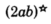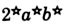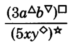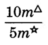$\frac{{4}^{3}{a}^{\Delta }{a}^{\square }}{4{a}^{\nabla }}$

${\left(\frac{4{x}^{\Delta }}{2{y}^{\nabla }}\right)}^{\square }$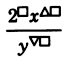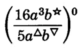## Exercises for review

( [link] ) Is there a smallest integer? If so, what is it?

no

( [link] ) Use the distributive property to expand $5a\left(2x+8\right)$ .

( [link] ) Find the value of $\frac{{\left(5-3\right)}^{2}+{\left(5+4\right)}^{3}+2}{{4}^{2}-2\cdot 5-1}$ .

147

( [link] ) Assuming the bases are not zero, find the value of $\left(4{a}^{2}{b}^{3}\right)\left(5a{b}^{4}\right)$ .

( [link] ) Assuming the bases are not zero, find the value of $\frac{36{x}^{10}{y}^{8}{z}^{3}{w}^{0}}{9{x}^{5}{y}^{2}z}$ .

$4{x}^{5}{y}^{6}{z}^{2}$

#### Questions & Answers

how can chip be made from sand
Eke Reply
is this allso about nanoscale material
Almas
are nano particles real
Missy Reply
yeah
Joseph
Hello, if I study Physics teacher in bachelor, can I study Nanotechnology in master?
Lale Reply
no can't
Lohitha
where is the latest information on a no technology how can I find it
William
currently
William
where we get a research paper on Nano chemistry....?
Maira Reply
nanopartical of organic/inorganic / physical chemistry , pdf / thesis / review
Ali
what are the products of Nano chemistry?
Maira Reply
There are lots of products of nano chemistry... Like nano coatings.....carbon fiber.. And lots of others..
learn
Even nanotechnology is pretty much all about chemistry... Its the chemistry on quantum or atomic level
learn
Google
da
no nanotechnology is also a part of physics and maths it requires angle formulas and some pressure regarding concepts
Bhagvanji
hey
Giriraj
Preparation and Applications of Nanomaterial for Drug Delivery
Hafiz Reply
revolt
da
Application of nanotechnology in medicine
has a lot of application modern world
Kamaluddeen
yes
narayan
what is variations in raman spectra for nanomaterials
Jyoti Reply
ya I also want to know the raman spectra
Bhagvanji
I only see partial conversation and what's the question here!
Crow Reply
what about nanotechnology for water purification
RAW Reply
please someone correct me if I'm wrong but I think one can use nanoparticles, specially silver nanoparticles for water treatment.
Damian
yes that's correct
Professor
I think
Professor
Nasa has use it in the 60's, copper as water purification in the moon travel.
Alexandre
nanocopper obvius
Alexandre
what is the stm
Brian Reply
is there industrial application of fullrenes. What is the method to prepare fullrene on large scale.?
Rafiq
industrial application...? mmm I think on the medical side as drug carrier, but you should go deeper on your research, I may be wrong
Damian
How we are making nano material?
LITNING Reply
what is a peer
LITNING Reply
What is meant by 'nano scale'?
LITNING Reply
What is STMs full form?
LITNING
scanning tunneling microscope
Sahil
how nano science is used for hydrophobicity
Santosh
Do u think that Graphene and Fullrene fiber can be used to make Air Plane body structure the lightest and strongest. Rafiq
Rafiq
what is differents between GO and RGO?
Mahi
what is simplest way to understand the applications of nano robots used to detect the cancer affected cell of human body.? How this robot is carried to required site of body cell.? what will be the carrier material and how can be detected that correct delivery of drug is done Rafiq
Rafiq
if virus is killing to make ARTIFICIAL DNA OF GRAPHENE FOR KILLED THE VIRUS .THIS IS OUR ASSUMPTION
Anam
analytical skills graphene is prepared to kill any type viruses .
Anam
Any one who tell me about Preparation and application of Nanomaterial for drug Delivery
Hafiz
what is Nano technology ?
Bob Reply
write examples of Nano molecule?
Bob
The nanotechnology is as new science, to scale nanometric
brayan
nanotechnology is the study, desing, synthesis, manipulation and application of materials and functional systems through control of matter at nanoscale
Damian
how did you get the value of 2000N.What calculations are needed to arrive at it
Smarajit Reply
Privacy Information Security Software Version 1.1a
Good
Berger describes sociologists as concerned with
Mueller Reply
what is hormones?
Wellington
Please keep in mind that it's not allowed to promote any social groups (whatsapp, facebook, etc...), exchange phone numbers, email addresses or ask for personal information on QuizOver's platform.
QuizOver Reply

### Read also:

#### Get Jobilize Job Search Mobile App in your pocket Now!

Source:  OpenStax, Elementary algebra. OpenStax CNX. May 08, 2009 Download for free at http://cnx.org/content/col10614/1.3
Google Play and the Google Play logo are trademarks of Google Inc.

Notification Switch

Would you like to follow the 'Elementary algebra' conversation and receive update notifications?ByByBy Cath YuBy IES PortalBy Yasser IbrahimBy Sam LuongBy Richley CrapoBy Saylor FoundationBy Stephen VoronBy Kevin MoquinBy Ellie BanfieldBy Rhodes